# 学妹和我讨论Py编程题后，我决定入坑LeetCode

### 学妹和我讨论Py编程题后，我决定入坑LeetCode

•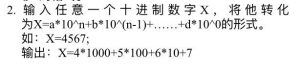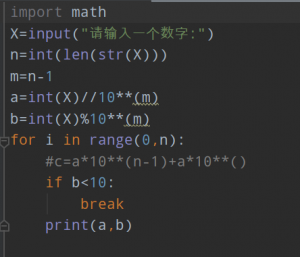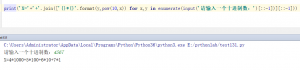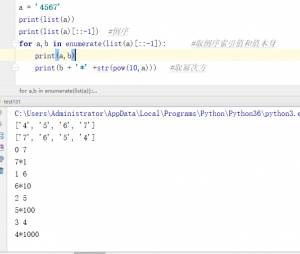enumerate() 函数用于将一个可遍历的数据对象(如列表、元组或字符串)组合为一个索引序列，同时列出数据和数据下标，一般用在 for 循环当中。

```X = input('请输入一个十进制数：')
result = []
for a,b in enumerate(X[::-1]):
result.append('{}*{}'.format(b,pow(10,a)))
print('X={}'.format('+'.join(result[::-1])))```
•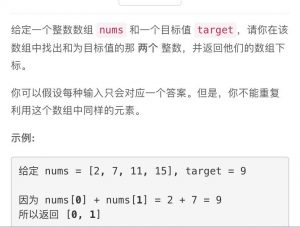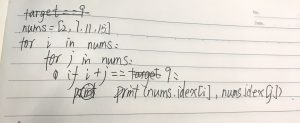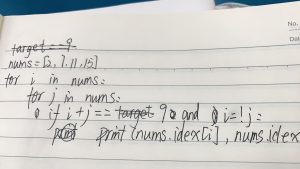•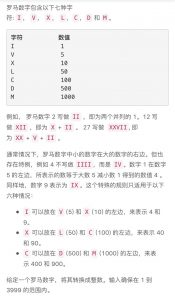VIII（8）可以看做V+I+I+I相加

```num = input('请输入一个罗马数字(介于1-3999之间)：')
nums = {'I':1,'V':5,'X':10,'L':50,'C':100,'D':500,'M':1000}
special ={'IV':4,'IX':9,'XL':40,'XC':90,'CD':400,'CM':900}
n = []
for i in special.items():
if i in num:
num = num.replace(i, '')
n.append(i)
for i in num:
n.append(nums[i])
print(sum(n))```

•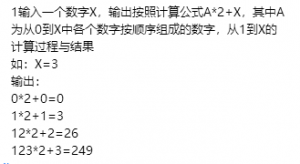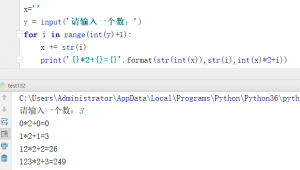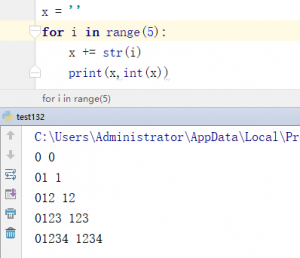•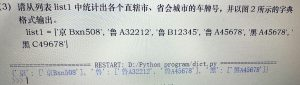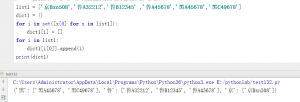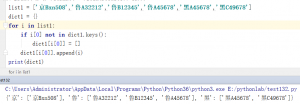•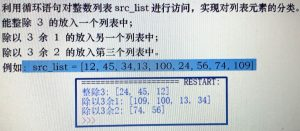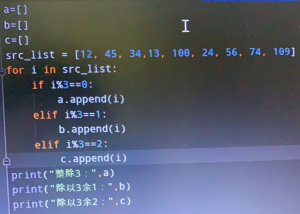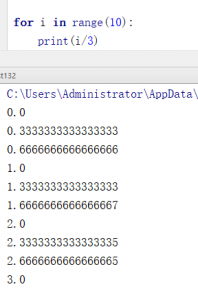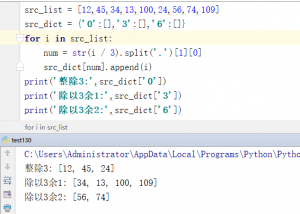微信赞赏支付宝赞赏